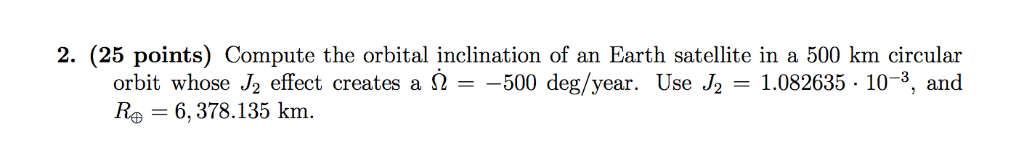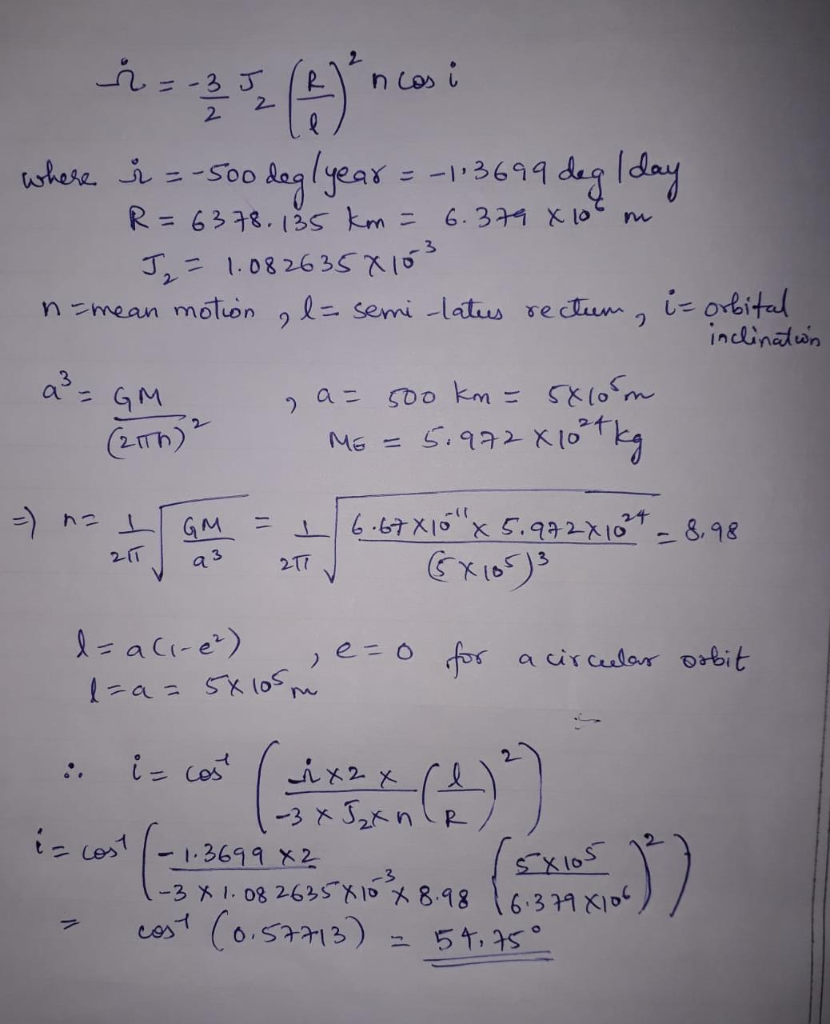# 2. (25 points) Compute the orbital inclination of an Earth satellite in a 500 km circular orbit w...2. (25 points) Compute the orbital inclination of an Earth satellite in a 500 km circular orbit whose J2 effect creates a 2-500 deg/year. Use J2 1.082635 - 10-3, and Re = 6,378.135 km.##### Add Answer of: 2. (25 points) Compute the orbital inclination of an Earth satellite in a 500 km circular orbit w...
More Homework Help Questions Additional questions in this topic.

• #### Assume a low Earth orbit (LEO) remote sensing satellite making observations is at an altitude of 500 km above the Earth¡¦s surface

Need Online Homework Help?